DCP HOME

# Laminar M-Convex Minimization

A laminar family means a nonempty family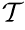of subsets of {1, ... , n} such that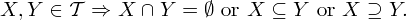For a laminar familyand a family of univariate discrete convex functions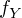indexed by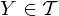, the function defined by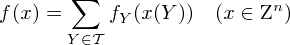is an M-convex function [1,2], where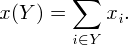Here we consider the following M-convex functions for laminar:

• quadratic laminar M-convex function: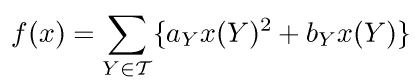• quartic laminar M-convex function:• exponential laminar M-convex function:• logarithmic laminar M-convex function: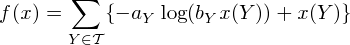This web application minimizes f(x) using ODICON.

 K. Murota (2001): "Discrete Convex Analysis---An Introduction (in Japanese)," Kyoritsu Publishing Company, Tokyo. Section 4.3.

 K. Murota (2003): "Discrete Convex Analysis," SIAM. Section 6.3.

 Satoko Moriguchi(satoko(a)aiit.ac.jp) Replace '(a)' with '@' modified on 5/4 0:05, 2012